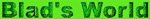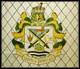Mathcad and Calculus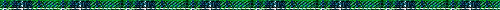Mathcad is a software program written by Mathsoft. It is a program that allows students to type formulas onto a screen in much the same way as using a word processor. The Mathcad software can solve, and graph these equations quickly and automatically. Mathcad is capable of doing most Calculus operations such as differentiation, integration, and even solving differential equations. Graphical capabilities include 2 and 3 dimensional plots as well as animation.   The key feature of Mathcad is that the screen is "live". That means students can change parameters within the equations and solutions as well as graphs change immediately.

Mathcad SitesCalculus Worksheets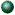Mathsoft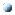Studyworks OnlineOld Dominion U.Old Dominion U.Numerical MethodsCalculus Animations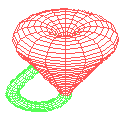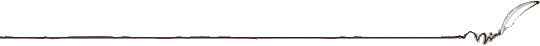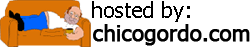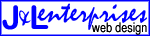Kris Lindeblad Better living ... through integration